# 算法的时间复杂度分析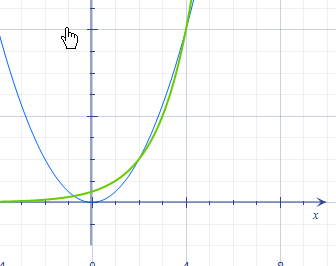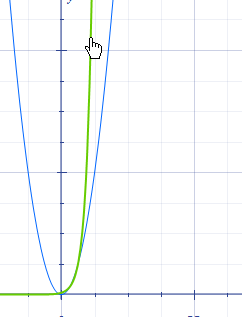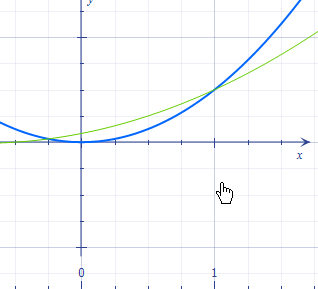N   1      2

c   >=6    >=3.75  .......

f(n)=O(g(n))  g(n)=O(h(n)))   ===>  f(n)=O(h(n))，而g(n)的时间复杂度就等于它本身的时间复杂度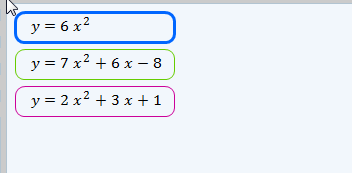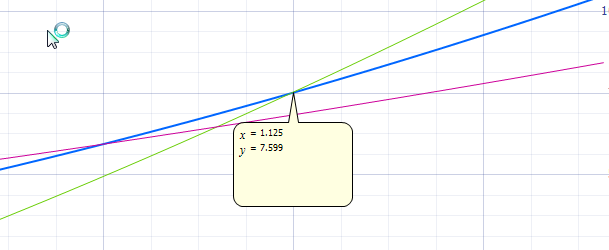f(n)=2n^2+3n+1

g(n)=n^2

h(n)=n^2

ok,那么我们现在知道了几个条件

c1=6 N1=1   c2=7 N2=0

n^k<=n^k+j  当c=1   ==》  N=1，这个关系就满足了，当N>1,g(n)函数就远远大于f(n)

性质5，如果f(n)=cg(n),那么f(n)=O(g(n));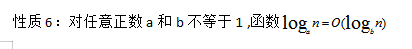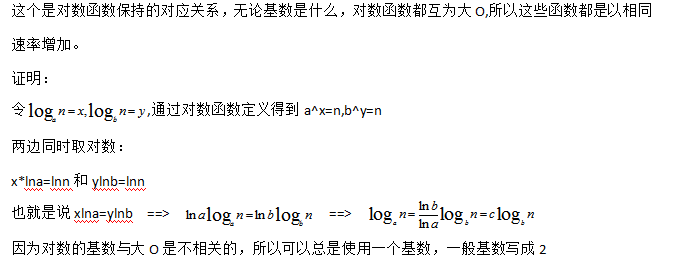08-142万+03-0543
03-23254
07-09564
11-104万+
04-17354
07-037001
04-12174
03-2367
05-19
12-20
09-18
01-157135
01-151万+